# All commands, alphabetic order; letter O

## \o (constant)

The \o command translates to a letter o with stroke, ø or &#xF8;. See description of the latin supplement characters.

## \O (constant)

The \O command translates to a capital letter o with stroke, Ø or &#xD8;. See description of the latin supplement characters.

## \oast (math symbol)

The \oast command is an alias for \circledast (Unicode U+229B; ⊛).

## \obar (constant)

The \obar command is valid only in math mode. It generates <mo>&omid;</mo> (Unicode U+29B6, ⦶).

## \obeylines

This commands makes the end-of-line character (control-M) active and behave like \par.

## \obeyspaces

This commands makes the space character active.

## \oblong (math symbol)

The \oblong command is an alias for \square (Unicode U+25A1, □).

## \ocircle (math symbol)

The \ocircle command is an alias for \circledcirc (Unicode U+229A, ⊚) (a circle in a circle).

## \oddsidemargin (rigid length)

This is a dimension, defined by LaTeX, but unused by Tralics.

## \odot (math symbol)

The \odot command is valid only in math mode. It generates a binary operator: <mo>&odot;</mo> (Unicode U+2299, ⊙). See description of the \pm command.

## \oe (constant)

The \oe command translates to an oe ligature (character U+153, œ). See description of the extended latin characters.

## \OE (constant)

The \OE command translates to an uppercase oe ligature (Unicode U+152, Œ) See description of the extended latin characters.

## \of

The \of command is undefined. However you say \root 3 \of 4 if you do not like the LaTeX syntax \sqrt{4}.

## \offinterlineskip

The \offinterlineskip command is ignored. In LaTeX, it sets \prevdepth to a magic value that inhibits insertion of interline glue, it sets \lineskip to zero and \lineskiplimit to the maximum possible value.

## \og (constant)

The \og command stands for ouvrez-les-guillemets. It is more or less the same as «. Translation is &#xAB;&#xA0;. For more info see the latin supplement characters.

## \ogreaterthan (math symbol)

The \ogreaterthan command is valid only in math mode. Its translation is <mo>&ogt;</mo> (Unicode U+29C1, ⧁).

## \oint (constant)

The \oint command is valid only in math mode. Its translation is a variable-sized symbol: <mo>&oint;</mo> (Unicode U+222E, ∮). For an example see the \sum command.

## \oldstylenums

The \oldstylenums command takes an argument and puts it in a <hi> element.

\begin{center}
1234567890 \\
\oldstylenums{1234567890}
\end{center}

<p rend='center'>1234567890 </p>
<p rend='center'><hi rend='oldstyle'>1234567890</hi></p>


The preview might be the following. This is not perfect: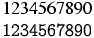.

## \olessthan (math symbol)

The \olessthan command is valid only in math mode. Its translation is <mo>&olt;</mo> (Unicode U+29C0, ⧀).

## \omega (math symbol)

The \omega command is valid only in math mode. It generates a Greek letter: <mi>&omega;</mi> (Unicode U+3C9, ω). See description of the \alpha command.

## \Omega (math symbol)

The \Omega command is valid only in math mode. It generates an uppercase Greek letter: <mi>&Omega;</mi> (Unicode U+3A9, Ω). See description of the \alpha command.

## \ominus (constant)

The \ominus command is valid only in math mode. It generates a binary operator: <mo>&ominus;</mo> (Unicode U+2296, ⊖). See description of the \pm command.

## \omit

The \omit command has as effect to omit the template of a cell in a table. This command is a primitive for \halign. For the tabular environment, you should use \multicolumn{1}{xx}{yy} instead, where xx is the desired template and yy the text.

Since \halign is not implemented, the \omit command provokes an error in the current version of Tralics.

## \omitcite [Tralics command]

The effect of \omitcite{something} is to omit the reference given as arguemnt from the bibliography. It is useful in the case of \nocite{*}. For further details, see the omitcite.tex file is the Tralics sources.

## \@ne

This command is made equal to the integer one, via \chardef (use it only in contexts where an integer is expected). For an example, see \m@ne.

## \onecolumn

This command is currently ignored.

## \on@line

The \on@line command can be used when signaling error; its expansion could be on input line 17.

## \@onlypreamble

If you say \@onlypreamle{\foo}, then the \begin{document} modifies the definition of \foo, provoking an error: Can be used only in preamble.

## \openin

You can say \openin 5=name, where the number 5 could be replaced by any number between 0 and 15, the equals sign is optional, and the file name is scanned like \input (the TeX primitive).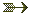(see scanint for details of argument scanning) (See also \input).

If a file is associated to the channel, it will be closed (in the same fashion as by \closein). Nothing special happens if the file cannot be opened. Otherwise, you can use \read to read from the the file associated to the channel.

Let's assume that the file tortureaux.tex contains the following six lines (the second and the last lines are blank).

abc

\a \b {\c
{} \d} \e
123



The following code assume that \testeq XY will signal an error if arguments are not equal. Note that the second \read reads the empty line; it is really empty because \endlinechar is not an integer between 0 and 216-1. The \read that follows will read two lines, because braces have to match.

{
\openin 5=tortureaux
\endlinechar=-1
\read 5 to \foo\testeq\foo{\a\b{\c{} \d} \e}
\closein5\relax
}\testeq\foo{123}


## \openout

You can say \openout 5=name, where the number 5 could be replaced by any number between 0 and 15 (see scanint for details). This closes any file attached to the output channel 5. It opens the file name for output (if the file exists, it content will be lost). In case of success, it marks the output channel as open (otherwise, it is closed).

The channel can be closed by \closeout, and you can write on it via \write. These three commands are whatsits: objects that are not immediately evaluated, but only when the box in which they are contained is shipped out, unless they are preceded by \immediate, case where the action is immediate. In Tralics, the \shipout command is never called, so that these actions are always immediate.

One reason why \immediate is needed is so that \write5{\thepage} prints the right page number.

You can say \typeout{foo}, this is the same as \write 17{foo}.

If you say \write N{list}, all tokens in the list are expanded, and the token list is printed. In case N refers to a valid output channel (associated to a file), printing goes to the file associated to this channel. Otherwise, output goes to the user's transcript file and to the terminal. Output is restricted to the transcript file if N is negative.

In the case where N=18, and Tralics is called with the --shell-escape option, then output goes to a shell, and is executed as an external Unix program (this depends on the operating system).

## \operatorname

This command can be used in math mode only, and \operatorname{sin} has the same meaning as \sin. There are some restrictions on the arguments: only characters are allowed. Since the LaTeX companion cites an example of the form \operatorname{ess\,sup}, commands that produce a space are accepted; spaces are otherwise ignored. When you say \DeclareMathOperator{\Sin}{sin}, this defines a command whose meaning is \operatorname{sin}. These two commands accept a starred form, see example

\DeclareMathOperator{\Sin}{sin}
\DeclareMathOperator*{\Limsup}{lim \, sup}
$\Sin = \sin = \operatorname{sin}$
$\Limsup = \limsup = \operatorname*{lim\;sup}$


Translation

<formula type='inline'>
<math xmlns='http://www.w3.org/1998/Math/MathML'>
<mrow>
<mo form='prefix'>sin</mo>
<mo>=</mo>
<mo form='prefix'>sin</mo>
<mo>=</mo>
<mo form='prefix'>sin</mo>
</mrow>
[/itex]
</formula>
<formula type='inline'>
<math xmlns='http://www.w3.org/1998/Math/MathML'>
<mrow>
<mo movablelimits='true' form='prefix'>lim sup</mo>
<mo>=</mo>
<mo movablelimits='true' form='prefix'>lim sup</mo>
<mo>=</mo>
<mo movablelimits='true' form='prefix'>lim sup</mo>
</mrow>
[/itex]
</formula>


## \operatornamewithlimits

This is the same as \operatorname*, see above.

## \OptionNotUsed

This is the default handler for package and class options. In the case of a package, the option is ignored, in the case of a class the option is added to the list of global options. You can redefine the command if the default behavior is not adequate.

## \oplus (math symbol)

The \oplus command is valid only in math mode. It generates a binary operator: <mo>&oplus;</mo> (Unicode U+2295, ⊕). See description of the \pm command.

## \or

The command \or is usually used to separate clauses inside an \ifcase construct. It can be used as boolean connector inside conditionals defined by \ifthenelse. (See \if... for details).

## \OR

It can be used as boolean connector (equivalent to \or) inside conditionals defined by \ifthenelse.

## \oslash (math symbol)

The \oslash command is valid only in math mode. It generates a binary operator: <mo>&oslash;</mo> (Unicode U+2298, ⊘). See description of the \pm command.

## \otimes (math symbol)

The \otimes command is valid only in math mode. It generates a binary operator: <mo>&otimes;</mo> (Unicode U+2297, ⊗). See description of the \pm command.

## \outer

You can say \outer\def\foo..... This defines the \foo command to be \outer, and this makes \foo behave like an end-of-file in many situations, for instance used as as argument of \xx, it will signal an error of the form Runaway argument? Forbidden control sequence \foo found while scanning use of \xx. A test done by \ifx is false if one command is \outer and the other is not.See also the \long command.

## \output

The result of \output={\foo}\the\output\the\output is the same \foo\foo. It is however unwise in TeX to use \output like this; in fact, whenever a page is full, TeX puts it in \box255 and evaluates the tokens of \output. After that, the box should be empty. On the other hand \deadcycles is increased (it is set to zero by \shipout). Since Tralics does not create pages of text, the output routine is never called, and \deadcycles is not modified.

## \outputpenalty (internal integer)

You can modify the \outputpenalty integer variable; however, TeX does not use it, it modifies it: it holds the value of the penalty that was at a page break (or 10000 otherwise). Unused by Tralics. Used a lot by LaTeX.(See scanint for details of argument scanning).

## \oval

You say \oval(dx,dy)[part] in order to put oval into a picture. See description of the picture environment for the syntax of the (dx,dy) part, and some examples. In general, the \oval is the argument of a \put(x,y){} command, and the (x,y) positions indicate the center of a rectangle, of size dx by dy, in which the oval should be drawn. If [part] is given, only part of the oval is drawn: the part can be t, b, l, r (top, bottom, left, right), or two such letters (tl means top left).

## \over

The \over command is a TeX primitive that should not be used in LaTeX. It is better to use \frac instead. We give here an example of \over and its variants. A construction like a \over b \over c is illegal in TeX. It generates an error in Tralics. In the case of {a \choose b} \quad {a\over b} \above1pt{c\over d} the numerator contains the \choose and the \over. This was not the intent of the author of the code. This is one reason why \over commands and such are discouraged. Example:

${a\over b}\quad {a\above1pt b}\quad {a\atop b}$
${a\overwithdelims() b}\quad {a\abovewithdelims()1pt b}% \quad {a\atopwithdelims() b}$

<formula type='inline'><math xmlns='http://www.w3.org/1998/Math/MathML'><mrow>
<mfrac><mi>a</mi> <mi>b</mi></mfrac>
<mspace width='1.em'/>
<mfrac linethickness='1pt'><mi>a</mi> <mi>b</mi></mfrac>
<mspace width='1.em'/>
<mfrac linethickness='0.0pt'><mi>a</mi> <mi>b</mi></mfrac>
</mrow>[/itex]</formula>
<formula type='inline'><math xmlns='http://www.w3.org/1998/Math/MathML'><mrow>
<mfenced open='(' close=')'><mfrac><mi>a</mi> <mi>b</mi></mfrac></mfenced>
<mspace width='1.em'/>
<mfenced open='(' close=')'><mfrac linethickness='1pt'><mi>a</mi> <mi>b</mi></mfrac></mfenced>
<mspace width='1.em'/>
<mfenced open='(' close=')'><mfrac linethickness='0.0pt'><mi>a</mi> <mi>b</mi></mfrac></mfenced>
</mrow>[/itex]</formula>


Preview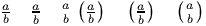(see also here)

## \overbrace

The \overbrace command puts an horizontal brace over a kernel. It works only in math mode. Example: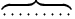.See also the \acute command.

## \overfullrule (rigid length)

You can say \overfullrule=5pt, as a result TeX will insert a rule of width 5pt at the end of each overfull rule. This is set to 0pt by LaTeX, ignored by Tralics.(See scandimen for details of argument scanning).

## \overleftarrow

The \overleftarrow command puts an arrow accent (Unicode character U+2190, ←) (pointing to the left) over a kernel. Example:. It works only in math mode.See also the \acute command.

## \overleftrightarrow

The \overleftrightarrow command puts an arrow accent (pointing to the left and right) over a kernel. (Unicode character U+2194, ↔) It works only in math mode.

## \overline

The \overline command puts a line over a kernel. It works only in math mode. See also the \acute command.

## \overrightarrow

The \overrightarrow command puts an arrow accent (Unicode character U+2192, →) (pointing to the right) over a kernel. Example: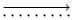. It works only in math mode. See also the \acute command.

## \overset

The \overset command takes two arguments A and B, it places A (superscript size) over B. It works only in math mode. See also the \acute command.

## \overwithdelims

The \overwithdelims command is a TeX primitive that should not be used. Instead of aa \overwithdelims()bb you should use \genfrac(){}{}{aa}{bb}.See \genfrac and \over.

## \owns (math symbol)

The \owns command is equivalent to \ni. It gives a normal symbol <mo>&ni;</mo> (Unicode U+220B, ∋). See also description of the \smallint command.back to home page © INRIA 2003-2005, 2006 Last modified $Date: 2017/05/29 06:22:57$